Sunday 25th July 2021# Classroom Key Stage 2 / KS2 - Maths Activities & Resources

## Welcome to the Maths section of the Key Stage 2 classroom. Within KS2 Maths you will find online @school assessment tests that will help children to enhance their learning and revise the subject of Maths at Key Stage 2 level.

•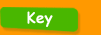•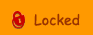••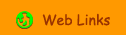•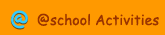•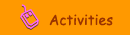•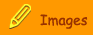###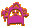Solving Problems

Boat Trip - Interactive test to calculate the different problems presented.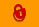Camping - Interactive test to solve various number problems.Charity - Test to solve a problem and give reasons for your answer.Coin Tossing - Interactive test about tossing coins.Cola Cans - Interactive test to solve a money problem.Counting Money - Interactive test to say what the different values of money are.Decimal Totals - Test to solve decimal problem.Jam - Interactive test about jam jars and money.Money 1 - Interactive money problem.Number Game - Interactive test to work out what the numbers are in the problem.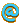Oranges - Interactive test about solving a numerical problem.Problem - 1 - Interactive test to work out which numbers can be divided by 9 remainder 1.Problem - 2 - Interactive test to find the height of one of the people displayed.Problem - 3 - Interactive test about calculating how many paper clips are made.Sports Day - Interactive test about solving numerical problems